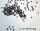# Iron sphere

Iron sphere has weight 100 kg and density ρ = 7600 kg/m3. Calculate the volume, surface and diameter of the sphere.

Result

V =  13.158 dm3
D =  2.929 dm
S =  26.953 dm2

#### Solution:

$m = 100 \ kg \ \\ h = 7600 \ kg/m^3 \ \\ m = hV \ \\ V_{ 1 } = \dfrac{ m }{ h } = \dfrac{ 100 }{ 7600 } = \dfrac{ 1 }{ 76 } \doteq 0.0132 \ m_{ 3 } \ \\ V = V_{ 1 } \rightarrow dm^3 = V_{ 1 } \cdot \ 1000 \ dm^3 = 13.1578947368 \ dm^3 = 13.158 \ dm^3$
$V = \dfrac{ 4 }{ 3 } \pi r^3 \ \\ r = \sqrt{ V \cdot \ \dfrac{ 3 }{ 4 \pi } } = \sqrt{ 13.1579 \cdot \ \dfrac{ 3 }{ 4 \cdot \ 3.1416 } } \doteq 1.4645 \ dm \ \\ D = 2 \cdot \ r = 2 \cdot \ 1.4645 \doteq 2.9291 = 2.929 \ \text { dm }$
$S = 4 \pi \cdot \ r^2 = 4 \cdot \ 3.1416 \cdot \ 1.4645^2 \doteq 26.9532 = 26.953 \ dm^2$

Leave us a comment of this math problem and its solution (i.e. if it is still somewhat unclear...):Be the first to comment!#### Following knowledge from mathematics are needed to solve this word math problem:

Do you want to convert length units? Do you know the volume and unit volume, and want to convert volume units?

## Next similar math problems:

1. Hollow sphereCalculate the weight of a hollow tungsten sphere (density 19.3 g/cm3), if the inner diameter is 14 cm and wall thickness is 3 mm.
2. SphereThe surface of the sphere is 12100 cm2, and weight is 136 kg. What is its density?
3. Hollow sphereSteel hollow sphere floats on the water plunged into half its volume. Determine the outer radius of the sphere and wall thickness, if you know that the weight of the sphere is 0.5 kg and density of steel is 7850 kg/m3
4. CubesOne cube is inscribed sphere and the other one described. Calculate difference of volumes of cubes, if the difference of surfaces in 257 mm2.
5. Sphere slicesCalculate volume and surface of a sphere, if the radii of parallel cuts r1=31 cm, r2=92 cm and its distance v=25 cm.
6. BallsPing pong balls have a diameter of approximately 5.1 cm. They are sold in boxes of 10 pieces: each box has the shape of a cuboid with a square base. The balls touch the walls of the box. Calculate what portion of the internal volume of the box is filled.
7. Spherical segmentSpherical segment with height h=6 has a volume V=134. Calculate the radius of the sphere of which is cut this segment.
8. Plasticine ballPlasticine balls have radius r1=85 cm, r2=60 mm, r3=59 cm, r4=86 cm, r5=20 cm, r6=76 mm, r7=81 mm, r8=25 mm, r9=19 mm, r10=14 cm. For these balls.
9. BallsThree metal balls with volumes V1=71 cm3 V2=78 cm3 and V3=64 cm3 melted into one ball. Determine it's surface area.
10. Sphere A2VSurface of the sphere is 241 mm2. What is its volume?
11. Cube in a sphereThe cube is inscribed in a sphere with volume 9067 cm3. Determine the length of the edges of a cube.
12. Spherical capFrom the sphere of radius 18 was truncated spherical cap. Its height is 12. What part of the volume is spherical cap from whole sphere?
13. Cube in ballCube is inscribed into sphere of radius 241 cm. How many percent is the volume of cube of the volume of sphere?
14. Sphere in coneA sphere of radius 3 cm desribe cone with minimum volume. Determine cone dimensions.
15. Two ballsTwo balls, one 8cm in radius and the other 6cm in radius, are placed in a cylindrical plastic container 10cm in radius. Find the volume of water necessary to cover them.
16. Shots5500 lead shots with diameter 4 mm is decanted into a ball. What is it diameter?
17. Sphere growthHow many times grow volume of sphere if diameter rises 10×?